# Field Functions Library (simion.experimental.field_array)¶

These are utility functions for conveniently representing vector fields from one or more potential array objects.

Motivation: A regular SIMION potential array represents a scalar field, where each point has a single value like potential V. It does not, however, by itself represent a vector field (like the electric field E or magnetic field B) which involves up to three values per point, one per each x, y, and z component of the field vector, except to the extent that these fields can be derived from the scalar field, like how SIMION knows that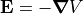. Not all fields, including certain magnetic fields, can be expressed in terms of a scalar field. One way around this is to store the field in three potentials arrays, one per scalar component of the field (e.g. “Bx.pa”, “By.pa”, and “Bz.pa”). This is certainly doable, but the question then is how to allow SIMION to properly interpret this data for the purposes of the Fly’m trajectory calculation, PE/Contour field visualization, and Data Recording, and that is where these functions come in handy. The PA’s can be wrapped in a “field array” object that provides functions for reading the vector field from the PA’s, applying it to the ion motion (e.g. mfield_adjust segment), passing it the contour plotting library, and other capabilities like computing a B field from a magnetic vector potential A stored in a field array object (curl operator). This library is especially useful for Magnetic Potential but is not limited to magnetic fields; any type of field can be represented.

For example, if you have a workbench with three potential array instances (“bx.pa”, “by.pa”, and “bz.pa”) representing the three components of a magnetic B-field, and they are numbered 1, 2, and 3 on PAs tab PA Instances list, then the B-field can be applied by creating a field array from the three potential arrays and building an mfield_adjust segment from it, as follows:

local B = simion.experimental.field_array{Bx=1,By=2,Bz=3}
print('TEST', B.bfield(10,20,0)) -- check a point


If, however, we have three potential array instances (“Ax.pa”, “Ay.pa”, “Az.pa”) representing a magnetic vector potential A, then we can equivalently apply the B field like this:

local A = simion.experimental.field_array{Ax=1,Ay=2,Az=3}
print('TEST', A.bfield(10,20,0))


Here is an example of applying a B-field from a single 2D cylindrical PA representing r A (for radial distance r and azimuthal component of magnetic vector potential A):

local rA = simion.experimental.field_array{rA=1}
print('TEST', rA.bfield(10,20,0))


The function to construct the field array from PA objects is simion.experimental.field_array(). The field array object has various methods and properties such as for reading the field or creating an mfield_adjust segment as shown above.

They functions are only available in SIMION 8.2 and are subject to change. A preliminary version of this was in SIMION Example: field_array in SIMION 8.1. This library is used in SIMION Example: field_array and SIMION Example: magnetic_potential.

class simion.experimental.field_array()
fa = simion.experimental.field_array{[x]=xpa, [y]=ypa, [z]=zpa,
painst=painst, antimirror=antimirror, type=type}


Returns an object representing a vector field consisting of the three provided potential arrays for the x, y, and z components of a vector field.

• xpa, ypa, and zpa are PA objects for each scalar component of the vector field. Of omitted (nil) the component is assumed to be everywhere zero. The labels x, y, and z can be any of the following:

• 1, 2, 3 - for x, y, and z components of an arbitrary vector field. Also note that {=xpa, =ypa, =zpa} is identical syntax to {xpa, ypa, zpa} in Lua.
• 'V' - electric potential in V.
• 'Ax', 'Ay', 'Az' - for the x, y, and z components of magnetic vector potential A in gauss mm ng. ng is a unit-less scaling constant, often set to 1.
• 'rA' - for r*A, in a 2D cylindrical symmetry field, where r is radial distance and A is the azimuthal (perpendicular to the 2D plane) component of magnetic vector potential in units of gauss mm2 ng. In SIMION, 2D cylindrical arrays are drawn in the XY plane, so r corresponds to y and the azimuth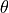corresponds to a z direction perpendicular to the XY plane.
• 'Bx', 'By', 'Bz' - for the x, y, and z components of the magnetic B-field in gauss.
• 'Ex', 'Ey', 'Ez' - for the x, y, and z components of the electric E-field in V/mm.

You may alternately specify here any PA instance objects on a workbench (e.g. simion.wb.instances) or, as a short-hand, PA instance numbers (e.g. 1).

If labels or type refers to magnetic potential or fields, then the PA objects must also be magnetic (pa.potential_type == 'magnetic').

• painst is an instance of a PA in workbench (e.g. simion.wb.instances). used only to translate between PA volume coordinates and workbench coordinates. It will will probably be, though not required to be, a PA instance of a PA object given above. Normally, this parameter is omitted (nil), in which case any PA instance object specified in the previous parameters is used, and if no PA instance was provided then no additional coordinate translation is done.

• antimirror is a string indicating any antimirror planes and may consist of any combination of the letters ‘x’, ‘y’, and ‘z’ (e.g. 'xz'). If omitted (nil), the default is '' for no antimirroring. Antimirroring means that the direction of the vector is reversed for negative values of the coordinate.

• type is the type of field to be represented:

• 'V' - electric potential V in Volts.
• 'A' - magnetic vector potential A in units of gauss mm ng.
• 'rA' - radial distance (r) times the aximuthal z component for magnetic vector potential, in a 2D cylindrical symmetry field, in units of of gauss mm2 ng.
• 'B' - magnetic B-field in gauss
• 'E' - electric E-field in V/mm

If omitted (nil), then the value is inferred from the labels above (e.g. 'Bx' implies 'B') or it remains undefined. The type can be required for some operations like fa.bfield.

Examples:

-- 3D vector field of magnetic vector potential A
local Axpa = simion.pas:open 'Ax.pa'
local Aypa = simion.pas:open 'Ay.pa'
local Azpa = simion.pas:open 'Az.pa'
local fa = simion.experimental.field_array {Axpa, Aypa, Azpa, type='A'}
local fa = simion.experimental.field_array {Ax=Axpa, Ay=Aypa, Az=Azpa} -- equivalent

-- 2D planar vector field of magnetic vector potential A_z
local Azpa = simion.pas:open 'Az.pa'
local fa = simion.experimental.field_array {nil, nil, Azpa, type='A'}
local fa = simion.experimental.field_array {Az=Azpa} -- equivalent

-- 2D cylindrical vector of r*A.
local rApa = simion.pas:open 'rA.pa'
local fa = simion.experimental.field_array {nil, nil, Azpa, type='rA'}
local fa = simion.experimental.field_array {rA=rApa} -- equivalent

-- Field with antimirror planes defined.
local fa = simion.experimental.field_array {Axpa,Aypa,Azpa, antimirror='xz'}

-- Field using PA instance objects or PA instance numbers.
local fa = simion.experimental.field_array {
Bx=simion.wb.instances, By=simion.wb.instances}
local fa = simion.experimental.field_array {Bx=1,By=2} -- equivalent

fa.field
f = fa.field
vx,vy,vz = f(x,y,z)


Returns a function f that can be used to obtain the field vector (vx,vy,vz) at any real valued point (x,y,z). f returns (0,0,0) outside of the PA volume.

If a PA instance was specified in the field_array constructor, then x, y, and z will be in mm in workbench coordinates; vx, vy, and vz will be oriented in workbench coordinates too; and the PA instance will be used to convert between workbench and PA volume coordinates. Otherwise, these variables are in mm (not gu) in PA volume coordinates. The units on vx, vy, and vz are whatever units are on the values stored in the underlying potential arrays.

One convenient use of f is to plot fields with the contourlib81 library (SIMION Example: contour). See SIMION Example: magnetic_potential for examples.

fa.grad
f = fa.grad
vx,vy,vz = f(x,y,z)


This is similar to fa.field but returns a vector that is the gradient of the represented field. For PA’s of type A (storing gauss mm ng), the units on the grad are gauss. For PA’s storing electric potential in V, the units on the grad are V/mm.

This assumes that the field array only contains a single scalar.

fa.curl
f = fa.curl
vx,vy,vz = f(x,y,z)


This is similar to fa.field but returns a vector that is the curl of the represented field. For PA’s of type A (storing gauss mm ng), the units on the curl are gauss. For PA’s storing electric potential in V, the units on the curl are V/mm.

One application of this function is if fa represents a vector magnetic potential, then f will represent the magnetic field since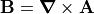. However, it is suggested to instead use the more general property fa.bfield instead.

fa.div
f = fa.div
vx,vy,vz = f(x,y,z)


This is similar to fa.field but returns a vector that is the divergence of the represented field. For PA’s of type A (storing gauss mm ng), the units on the divergence are gauss. For PA’s storing electric potential in V, the units on the divergence are V/mm.

[TODO: This function is not currently implemented.]

fa.bfield
f = fa.bfield
Bx,By,Bz = f(x,y,z)


This returns a B field of the given field array. This is similar to fa.field except that the returned vector is a B field in gauss. If the field array represents 'A' or 'rA' field type (magnetic vector potential), it will be re-expressed as a 'B' field type (e.g. byusing fa.curl) and returned. An error will be raised if it is not known how to express the field as a B field.

fa.efield
f = fa.efield
Ex,Ey,Ez = f(x,y,z)


This returns a E field of the given field array. This is similar to fa.field except that the returned vector is a E field in V/mm. If the field array represents 'V' field type (scalar electric potential in Volts), it will be re-expressed as a 'E' field type (e.g. byusing fa.grad) and returned. An error will be raised if it is not known how to express the field as a E field.

fa.efield_adjust
segment.efield_adjust = fa.efield_adjust


This returns an efield_adjust user program segment that represents the E field in the given field array object. It can be assigned to or called from segment.efield_adjust in a workbench user program. This function in fact is equivalent to

local efield_adjust = simion.experiment.make_efield_adjust(fa.efield)

fa.mfield_adjust
segment.mfield_adjust = fa.mfield_adjust


This returns an mfield_adjust user program segment that represents the B field in the given field array object. It can be assigned to or called from segment.mfield_adjust in a workbench user program. This function in fact is equivalent to

local mfield_adjust = simion.experiment.make_mfield_adjust(fa.bfield)

fa:refine()
fa:refine { . . . }


Refines all three potential arrays in the vector field. This supports the same parameters as the simion.pas pa:refine() function for regular potential arrays. One difference, however, is that the charge field, if provided, must be another vector field object returned by simion.experimental.field_array().

A typical example is this:

local Afa = simion.experimental.field_array{Axpa, Aypa, Azpa}
-- magnetic vector potential field
local jfa = simion.experimental.field_array{jxpa, jypa, jzpa}
-- current density vector field
Afa:refine { charge=jfa, convergence=1e-5 }
-- Poisson solve both together.

fa:save()
fa:save( [filename] )


Saves all three potentials arrays in the vector field. This is similar to the simion.pas pa:save() function for regular potential arrays. It will append ‘x’, ‘y’, or ‘z’ to the base of the specified filename. For example, fa:save('A.pa') will actually create the files ‘Ax.pa’, ‘Ay.pa’, and ‘Az.pa’. If filename is omitted, the existing file names will be used.

simion.experimental.make_efield_adjust()
Ex,Ey,Ez = efield(x,y,z)


Creates an efield_adjust segment for a workbench user program given a field function efield that inputs a point in workbench coordinates and returns the E field vector components at that point in units of V/mm and oriented in workbench coordinates. Example:

local function efield(x,y,z) return 10,20,0 end  -- V/mm


The returned function is equivalent to

function segment.efield_adjust()
local inst = simion.wb.instances[ion_instance]
local dx_mm = inst.scale*inst.pa.dx_mm
local dy_mm = inst.scale*inst.pa.dy_mm
local dz_mm = inst.scale*inst.pa.dz_mm
local Ex,Ey,Ez = efield(ion_px_mm,ion_py_mm,ion_pz_mm)  -- -grad V (V/mm)
Ex,Ey,Ez = -Ex*dx_mm,-Ey*dy_mm,-Ez*dz_mm                --  grad V (V/gu)
ion_dvoltsx_gu,ion_dvoltsy_gu,ion_dvoltsz_gu =
wb_orient_to_pa_orient(Ex,Ey,Ez)
end


See also fa.efield_adjust.

simion.experimental.make_mfield_adjust()
Bx,By,Bz = bfield(x,y,z)


Creates an mfield_adjust segment for a workbench user program given a field function bfield that inputs a point in workbench coordinates and returns the B field vector components at that point in units of gauss and oriented in workbench coordinates. Example:

local function bfield(x,y,z) return 10,20,0 end  -- gauss


The returned function is equivalent to

function segment.mfield_adjust()
ion_bfieldx_gu,ion_bfieldy_gu,ion_bfieldz_gu =
wb_orient_to_pa_orient(bfield(ion_px_mm,ion_py_mm,ion_pz_mm))
end


See also fa.mfield_adjust.

simion.experimental.bfield_of_scalar()
bfield = simion.experimental.bfield_of_scalar(painst, mu, antimirror)
Bx,By,Bz = bfield(x,y,z)


Returns function f representing B-field given PA instance object (painst) of magnetic scalar potential and relative permeability mu. mu is a function, PA object, PA instance object, or nil (relative permeability defaults to 1 if nil). f maps point (x,y,z) in workbench coordinates (mm) to vector (Bx,By,Bz) in gauss.

Warning: this function is subject to change.

simion.experimental.spotential_from_vfield()
simion.experimental.spotential_from_vfield(painst, vfield [, N])


Fills PA instance painst with scalar potentials based on vector field given in vfield. We assume the vector field is the negative gradient of the scalar potential. If painst is electric, representing electric potential (V), then vfield represents the E-field. If painst is magnetic, representing magnetic scalar potential (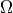), then vfield represents the H-field. vfield is a function of the form vx,vy,vz = vfield(x,y,z) where (x,y,z) is a point in workbench coordinates (mm). If painst is electric, then vfield should be in units of V/mm. If painst is magnetic, then vfield should actually be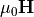, which, like B, has units of gauss, and it equals B in the case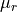= 1.

Point (0,0,0) is arbitrarily assigned potential 0.

The given vector field must actually be representable in terms of scalar magnetic potential; otherwise, results will be nonsensical. In particular, if the PA instance is magnetic, the volume of the PA should not contain currents, or at least currents significant enough to generate magnetic fields. If you have currents, you may break up your PA into smaller PAs that are current free.

This function is similar to the pa field field setting function in the older SL Libraries and the “Text to PA” function in SL Tools. This is a rudimentary line integration based on the trapezoidal rule, though it averages over multiple paths (x, y, and z directions) to improve accuracy.

N is the number of steps per grid point in the integration. The default (if omitted) is 1. Higher values increase accuracy.

simion.experimental.make_cell_scalar()
scalar = simion.experimental.make_cell_scalar(painst, pa)
v = scalar(x,y,z)


Returns function scalar representing values in PA object pa. pa if omitted defaults to painst.pa where painst is a PA instance object. scalar has the form v = scalar(x,y,z) where (x,y,z) are in workbench coordinates. PA grid point (x,y,z) is assumed to represent region (x,y,z) inclusive to (x+1,y+1,z+1) exclusive. Therefore, this function may be used for things like charge density and permeability where values in the PA object represent the average value in the entire cell. This function should not be used for things like electric potential where values in the PA object represent the value at the cell vertex. Returns nil if point outside array (TODO: subject to change).

Warning: this function is subject to change.

# TODO¶

• nz_use on 2D planar arrays is currently ignored in checking if point is within volume.

# Changes/History¶

• 8.2EA 8.1.2.7 2014-03-14: Expanded library, simplified and much changed API, and renamed to simion.experimental.field_array.
• 8.2EA 8.1.2.7 2014-03-07: Fixed handling of cylindrical magnetic vector potential(function previously named bfield_vector). B-field component in Y direction (By) should have been divided by psipa.dy_mm (grid cell Y size in mm). The example mu_sphere_2dc-Az.gem had been improperly scaling magnetic vector potential to correct for this error. Now also returns 0,0,0 outside the array.
• Based on the maglib library (2012-04-30) in an older version of SIMION Example: magnetic_potential (8.2EA) See the README of that example for older changes.
• Based partly on the fieldlib library SIMION Example: field_array in SIMION 8.1.
• Inspired partly from the simionx.FieldArray - 3D vector field library in SIMION 8.0.